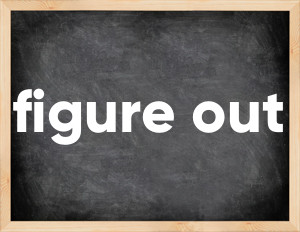# Phrasal verb figure outThe English verb 'figure out' is pronounced as [ˈfɪgə aʊt].
Related to: regular verbs, phrasal verb.
3 forms of verb figure out: Infinitive (figure out), Past Simple - (figured out), Past Participle - (figured out).

## Here are the past tense forms of the verb figure out

👉 Forms of verb figure out in future and past simple and past participle.
❓ What is the past tense of figure out.

## Figure out: Past, Present, and Participle Forms

Base Form Past Simple Past Participle
figure out [ˈfɪgə aʊt]

figured out [fɪgəd aʊt]

figured out [fɪgəd aʊt]

## What are the 2nd and 3rd forms of the verb figure out?

🎓 What are the past simple, future simple, present perfect, past perfect, and future perfect forms of the base form (infinitive) 'figure out'?

### Learn the three forms of the English verb 'figure out'

• the first form (V1) is 'figure out' used in present simple and future simple tenses.
• the second form (V2) is 'figured out' used in past simple tense.
• the third form (V3) is 'figured out' used in present perfect and past perfect tenses.

## What are the past tense and past participle of figure out?

The past tense and past participle of figure out are: figure out in past simple is figured out, and past participle is figured out.

### What is the past tense of figure out?

The past tense of the verb "figure out" is "figured out", and the past participle is "figured out".

### Verb Tenses

Past simple — figure out in past simple figured out (V2).
Future simple — figure out in future simple is figure out (will + V1).
Present Perfect — figure out in present perfect tense is figured out (have/has + V3).
Past Perfect — figure out in past perfect tense is figured out (had + V3).

### figure out regular or irregular verb?

👉 Is 'figure out' a regular or irregular verb? The verb 'figure out' is regular verb.

## Examples of Verb figure out in Sentences

•   Can you figure out this word? (Present Simple)
•   It's hard to read the feelings of others when you still haven't figured out your own (Present Perfect)

• Figure out мы используем, когда получаем информацию при помощи умственной деятельности, логики, анализа и рассуждений.
• Find out мы используем, когда подразумеваем, что информация поступает извне, то есть человек просто ее получает. Почти всю информацию мы получаем при помощи зрения и слуха, то есть, вы можете услышать её от кого-то, или прочитать, увидеть.

Along with figure out, words are popular break in and loiter.

Verbs by letter: , , , , , , , , , , , , , , , , , , , , , , , , .